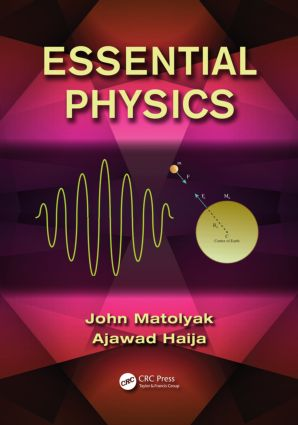Essential Physics

1st Edition

CRC Press

472 pages | 438 B/W Illus.

Purchasing Options:\$ = USD
Hardback: 9781466575219
pub: 2013-12-17
SAVE ~\$26.00
\$130.00
\$104.00
x
eBook (VitalSource) : 9780429101502
pub: 2013-12-17
from \$65.00

FREE Standard Shipping!

Description

Fluency with physics fundamentals and problem-solving has a collateral effect on students by enhancing their analytical reasoning skills. In a sense, physics is to intellectual pursuits what strength training is to sports.

Designed for a two-semester algebra-based course, Essential Physics provides a thorough understanding of the fundamentals of physics central to many fields. It omits material often found in much larger texts that cannot be covered in a year-long course and is not needed for non-physics majors. Instead, this text focuses on providing a solid understanding of basic physics and physical principles. While not delving into the more specialized areas of the field, the text thoroughly covers mechanics, electricity and magnetism, light, and modern physics.

This book is appropriate for a course in which the goals are to give the students a grasp of introductory physics and enhance their analytical problem-solving skills. Each topic includes worked examples. Math is introduced as necessary, with some applications in biology, chemistry, and safety science also provided. If exposure to more applications, special topics, and concepts is desired, this book can be used as a problem-solving supplement to a more inclusive text.

Systems of Units, Significant Figures, Coordinate Systems, and Vectors

Systems of Units

Conversion of Units

Significant Figures

Scientific Notation

Trigonometry

Coordinate Systems

Vectors

Addition and Subtraction of Vectors

Guideline for Determining the Direction of the Resultant

Motion in One Dimension

Displacement

Average Velocity

Average Speed

Instantaneous Velocity

Acceleration

Equations of Motion

Free-Falling Object

Two-Dimensional Motion and Circular Motion

Displacement, Velocity, and Acceleration

Equations of Motion in Two Dimensions

Projectile Motion

Uniform Circular Motion

Linear Velocity, Angular Velocity, Period, and Frequency

Nonuniform Circular Motion

Newton’s Laws: Implications and Applications

Statement of Newton’s Laws

Discussion of Newton’s Laws of Motion

Free Body Diagram

Remarks on the Application of Newton’s Second and Third Laws

Newton’s Gravitational Law

Mass, Weight, and Newton’s Gravitational Law

Newton’s Third Law and Apparent Weight

Dynamics of a Uniform Circular Motion

Newton’s Laws: Friction in Linear and Circular Motions

Frictional Forces

Work and Energy

Work

Work–Energy Theorem

Conservative Forces

Potential Energy

Nonconservative Forces and the Total Mechanical Energy

Power

Linear Momentum and Collision

Systems of Particles

Motion of the Center of Mass

Linear Momentum of a System of Particles

Collisions and Change in Linear Momentum

Impulse

Collisions in Two Dimensions

Types of Collision

Rotational Motion

Angular Kinematic Quantities

Rotational Motion in a Plane

Torque

Rigid Body

Moment of Inertia

Parallel Axis Theorem

Equilibrium of a Rigid Body

Angular Momentum

Rotational Kinetic Energy

A Rigid Body in Translational and Rotational Motions

Total Mechanical Energy of a Rigid Body

Simple Harmonic Motion

Hooke’s Law

Potential Energy of a Spring

Mass-Spring System in SHM

Simple Pendulum in SHM

Thermal Physics: Temperature, Heat, and Thermal Expansion

Heat is a Form of Energy, Specific Heat, and Heat Capacity

Heat and Internal Energy

Zeroth Law of Thermodynamics

Thermometers and Temperature Scales

Thermal Expansion

Calorimetry

Heat Transfer: Transfer by Conduction

Waves and Wave Motion

Wave Motion

Types of Waves

Mathematical Treatment of an Ideal Wave

Intensity of Waves

Superposition of Waves: Interference and Standing Waves

Fluids

Pressure: Definition and Units

Pressure Within Fluids

Density of Fluids

Variation of Pressure with Depth in a Static Fluid

Pascal’s Principle and Applications

Archimedes’ Principle

Dynamics of Fluids: Equation of Continuity

Bernoulli’s Equation

Electric Forces and Fields

Introduction

Conductors and Insulators: Charged Objects

Coulomb’s Law

The Electric Field

Conductors in an Electric Field

Gauss’ Law

Electric Potential Energy and Potential

Introduction

Potential Energy

Electric Potential

Electric Potential of Point charges: Spatially Varying E

Direct Current Circuits

Introduction

Ohm’s Law

Resistivity

Simple Circuits

Electric Power

Kirchhoff’s Rules

Capacitors

Magnetic Forces and Fields

Introduction

The Magnetic Field

The Magnetic Force on an Electric Current

Magnetic Fields Produced by Moving Charges

Magnetic Materials and Permanent Magnets

Electromagnetic Induction

Introduction

Electric Generators

Self-Inductance

Transformers

Alternating Current Electric Circuits

Introduction

Alternating Signals

Phase Relations in Simple AC Circuits

RCL Series Circuit

Root-Mean-Square Average Values, Power

Electromagnetic Waves

Introduction

The Electromagnetic Spectrum

Geometrical Optics

Introduction

Wavefronts and Rays

Reflection

Refraction

Thin Lenses

Optical Instruments

Physical (Wave) Optics

Introduction

Double-Slit Experiment

Thin-Film Interference

Single-Slit Diffraction

The Diffraction Grating

Polarization

Modern Physics

Introduction

Atoms

Line Spectra

The Bohr Model

Beyond the Bohr Model

The Periodic Table of Elements

X-Rays

Quantum Physics

Wave–Particle Duality

Quantum Mechanics

The Heisenberg Uncertainty Principle

The Atomic Nucleus, Radioactivity